# SAT II Math I : Range and Domain

## Example Questions

1 3 Next →

### Example Question #21 : Functions And Graphs

Find the domain and range of the function. Express the domain and range in interval notation.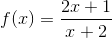### Domain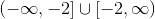### Range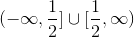### Domain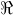(all real numbers)

### Range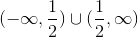### Domain### Range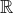(all real numbers)

##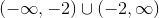### Range### Domain### Range##### RangeExplanation:Finding the Domain

The domain of a function is defined as the set of all valid input values ofoverwhich the function is defined. The simple rule of thumb for rational functions is that all real numbers will work except for those in which denominator is zero since division by zero is not allowed.

Set the denominator to zero and solve for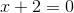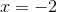The function is therefore defined everywhere except at. Therefore the domain expressed in interval notation is,Note that the open parentheses indicate that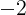is not in the domain, butmay become arbitrarily close to.

Finding the Range

The range of a function is defined as the set of all outputs spanning the domain. Finding the range can be achieved by finding the domain of the inverse function. First solve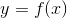forto obtain the inverse function,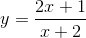Multiply both sides by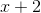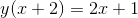Distribute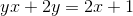Move all terms withto one side of the equation,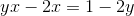Factor and solve for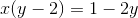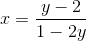The inverse function is therefore,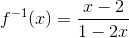Find the domain of the inverse function,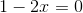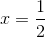The range ofis the domain of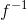, which is:If you look at the plots for the function(in blue) and(in red and labeled as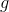in the figure) you can see the asymptotic behavior ofasapproachesand ofasapproaches.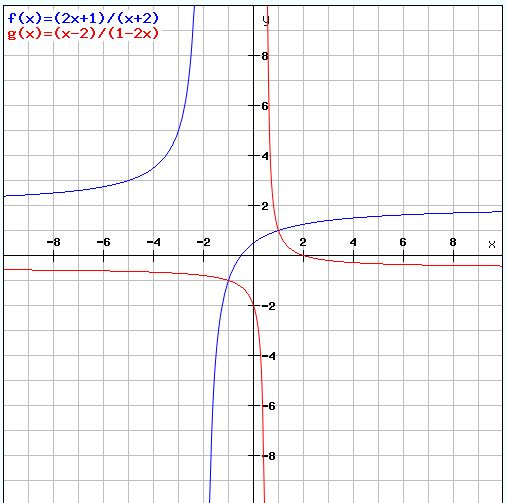### Example Question #22 : Functions And Graphs

Determine the domain for the function given: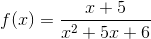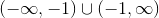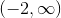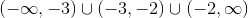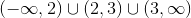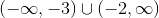Explanation:

To determine the domain of the function, we must ask ourselves where x can and cannot exist. On the numerator, nothing is preventing x from existing anywhere. But the denominator of the function cannot equal zero (which would produce an undefined value for the function), so to determine at which x values this occurs, we must set the denominator equal to zero and solve for x: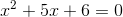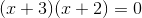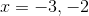(The factors of 6 that add up to 5 are 3 and 2.)

Because these are the only values that x equal for the function to exist, we make our intervals as shown below: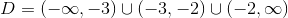We use round brackets to indicate that we never include the bounds of the intervals in the domain.

### Example Question #23 : Functions And Graphs

Find the domain of the function given: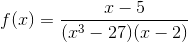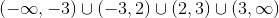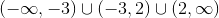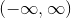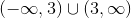Explanation:

To determine the domain of the function, we must consider where x cannot exist. The only limitation on the function is the denominator, which cannot equal zero.

To find the x-values where this occurs, we must set the denominator equal to zero and solve for x: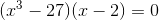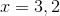These are the only two limitations on the domain of the function, so the domain of the function isNote that round brackets were used for all of the intervals, because none of the bounds of the intervals are included in the domain.

### Example Question #24 : Functions And Graphs

Find the domain of the given function: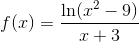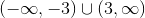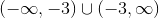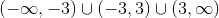Explanation:

To determine the domain of the function, we must find the x-values that would give us an undefined result when we plug them into the function. On the numerator, we know that the natural log function can never equal zero or be negative. In the denominator, we can never have a zero. With these conditions in mind, we must now find the x values that makes these undefined situations occur.

For the numerator: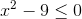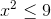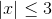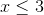and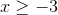For the denominator: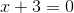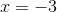Now that we know where x cannot be, we can now write the domain, making sure to use round brackets for the endpoints of the intervals:### Example Question #25 : Functions And Graphs

What is the domain of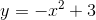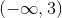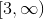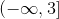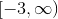The answer is: